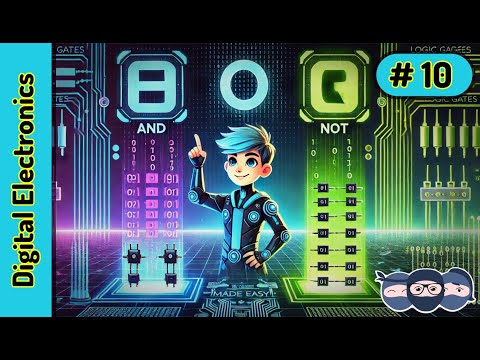# how to visualize electricity? (including question about logic cirquits)?

Ok so I have originally had a hard time visualizing electricity (volt, ampere, watt difference) and reading online it is often explained as a a stream of water (which also has pressure and a current) so far it seemed OK until i stumbled upon an explanation of logic cirquits.

To my understanding it is basically a boolean set of requirements that depending on the input determines the output. I understand that as "depending on the variables of electricity coming in or not, will determine whether electricity is allowed to go out or not"

Visualizing it as water, an AND circuit is only open, or "1" if both a and b pipes are open, or "1". So if water comes from pipe a but not from b, the circuit will return "0" and remain closed. So far so good.But then there are things like NAND circuits, basically returning the opposite value of an AND circuit.But if both a and b are at 0, meaning water isnt flowing, how can output be "1"? No water is coming in so it is not logical for there to be output. Visualizing this as electricity I dont understand the purpose of such circuits and encountering this problem I feel there is a fundamental misunderstanding in how electricity flows, or perhaps even in the purpose and function of logic circuits.Thanks for any answers.

Relevance

The water analogy is fine for electricity:

Volts being pressure

Current being the flow of water

Resistance being a constriction in the pipe which reduces flow.

Logic circuitry, throw away the water analogy. Logic is math (Boolean algebra) and flip-flops. That's an over simplification since actual logic circuits utilize active electronic components (diodes and transistors) and you must worry about current flow. When logic gets fast, you are actually designing circuity in the analog domain where resistance, capacitance, propagation time, reflections, meta stability all become issues.

•Login to reply the answers
• Forget about the water analogy, and just think of the truth table in terms of voltage levels. As it happens, there are several transistors inside an AND or NAND gate that, at least for MOS devices are essentially voltage activated. The transistors are connected in such a way that the gates conform to the truth table for the specified logic function (AND, OR, XOR, NOR, NAND, ETC)

So for a certain technology of transistors, a certain level of voltage (usually the supply voltage) is assigned to be a logic 1 (one) and another level, usually zero volts, is assigned to be a logic zero, or if you prefer true and false.

The purpose of these circuits is to implement the logic of the program written by the programmer (after it has been converted to machine language).

Hope this helps a little.

•Login to reply the answers
• You can use the water analogy for logic gates using the concept of a water pressure controlled tap.

So a regular tap has a lever to control on/off. Imagine a tap with a pipe inlet instead of a lever. Applying water pressure to that pipe controls whether water flows in other pipes.

A logic gate is an electrically controlled switch. What I've described above is a water controlled value which is equivalent.

•Login to reply the answers
• Do not try to visualize Boolean logic electrically, It will drive you crazy.

You can examine the IC's electrically but not Boolean logic. Boolean logic is simply 1's and 0's traveling through the IC's.

Much simpler.

•Login to reply the answers
•Login to reply the answers•Login to reply the answers